20张图揭开「队列」的迷雾，一目了然！## 队列的概念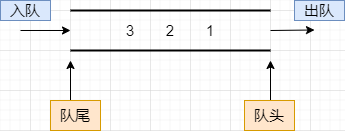### 我们可以总结如下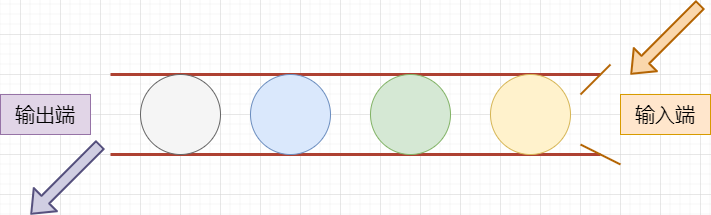### 队列存储结构的实现有以下两种方式

• 顺序队列：在顺序表的基础上实现的队列结构

• 链队列：在链表的基础上实现的队列结构

## 队列的结点设计与初始化next指针表示，下一个的指针，其指向下一个结点，通过next指针将各个结点链接。``````//结点定义
typedef struct node{
int data;
struct node *next;
}node;
//队列定义，队首指针和队尾指针
typedef struct queue{
node *front;    //头指针
node *rear;     //尾指针
}queue;
``````

``````//初始化结点
node *init_node(){
node *n=(node*)malloc(sizeof(node));
if(n==NULL){    //建立失败，退出
exit(0);
}
return n;
}

//初始化队列
queue *init_queue(){
queue *q=(queue*)malloc(sizeof(queue));
if(q==NULL){    //建立失败，退出
exit(0);
}
//头尾结点均赋值NULL
q->front=NULL;
q->rear=NULL;
return q;
}
``````

## 判断队列是否为空

``````//队列判空
int empty(queue *q){
if(q->front==NULL){
return 1;   //1--表示真，说明队列非空
}else{
return 0;   //0--表示假，说明队列为空
}
}
``````

``````int empty(queue *q){
return q->front==NULL;
}
``````

## 入队操作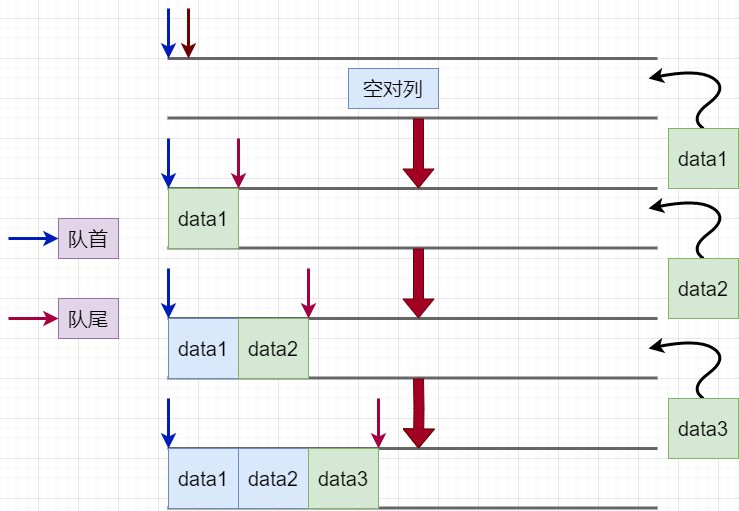``````front=n;
rear=n;
``````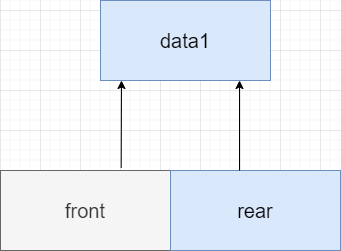唯一结点n``````//入队操作
void push(queue *q,int data){
node *n =init_node();
n->data=data;
n->next=NULL;   //采用尾插入法
//if(q->rear==NULL){     //使用此方法也可以
if(empty(q)){
q->front=n;
q->rear=n;
}else{
q->rear->next=n;    //n成为当前尾结点的下一结点
q->rear=n;  //让尾指针指向n
}
}
``````

## 出队操作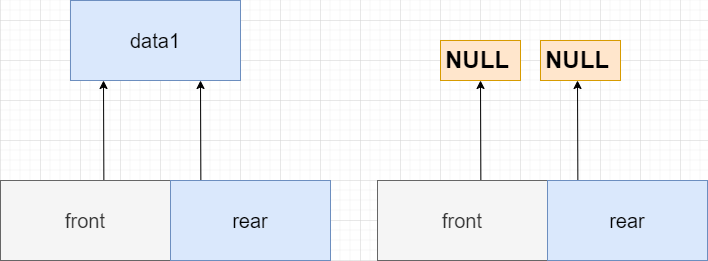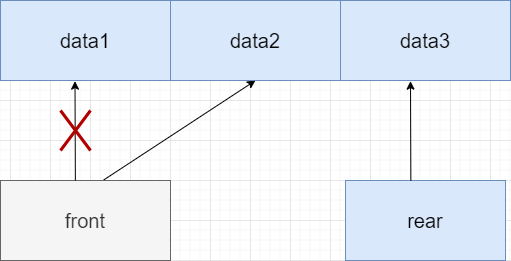``````//出队操作
void pop(queue *q){
node *n=q->front;
if(empty(q)){
return ;    //此时队列为空，直接返回函数结束
}
if(q->front==q->rear){
q->front=NULL;  //只有一个元素时直接将两端指向置为空即可
q->rear=NULL;
free(n);        //记得归还内存空间
}else{
q->front=q->front->next;
free(n);
}
}
``````

## 打印队列元素（遍历）

``````//打印队列元素
void print_queue(queue *q){
node *n = init_node();
n=q->front;
if(empty(q)){
return ;    //此时队列为空，直接返回函数结束
}
while (n!=NULL){
printf("%d\t",n->data);
n=n->next;
}
printf("\n");   //记得换行
}
``````

``````int calac(queue *q){
node *n = init_node();
n=q->front;
int cnt=0;    //计数器设计
if(empty(q)){
return 0;    //此时队列为空，直接返回函数结束
}
while (n!=NULL)
{
n=n->next;
cnt++;
}
return cnt;
}
``````

## 顺序队列的假溢出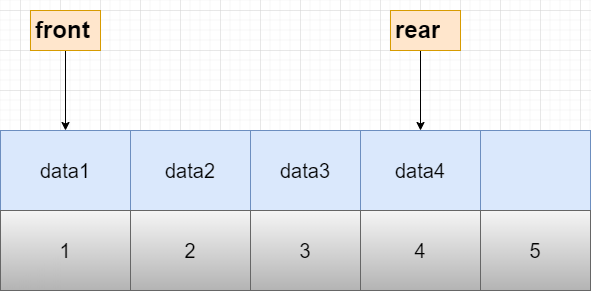示例顺序队列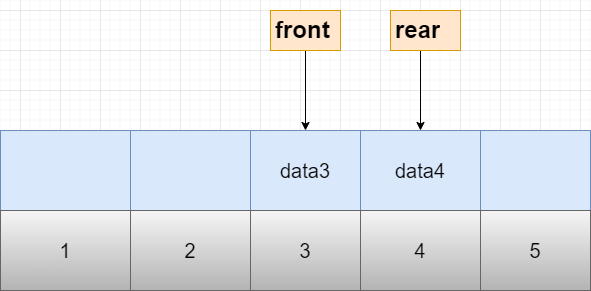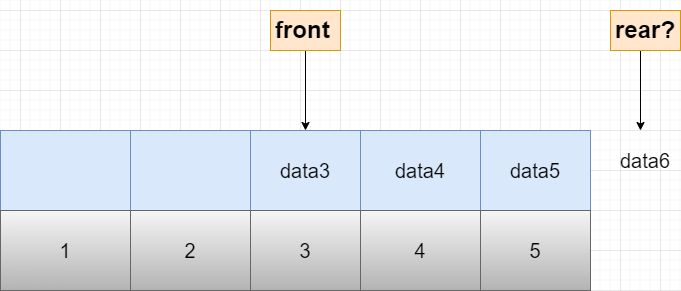出队产生假溢出

## 循环队列的概念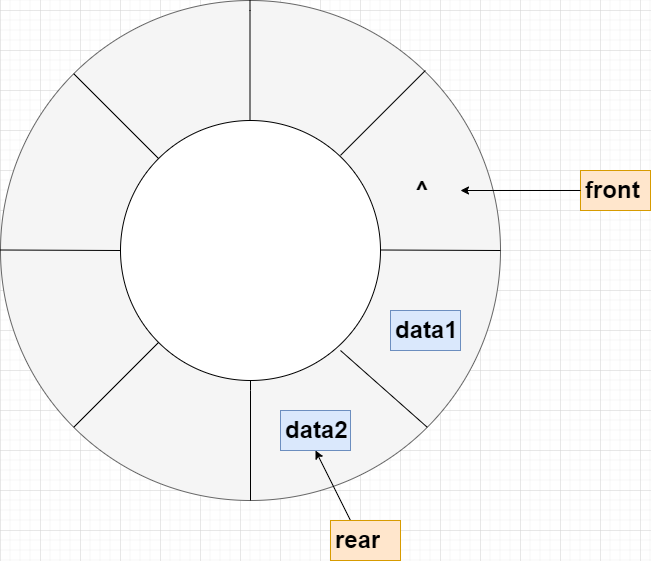## 循环队列的结构设计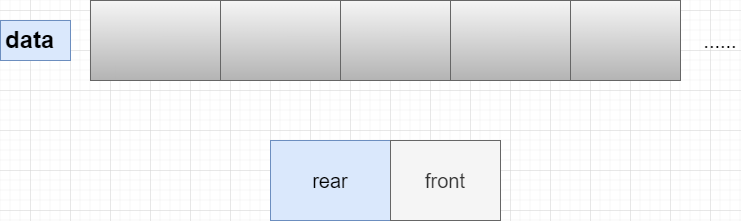• `maxsize`表示循环队列的最大容纳量，其表示队列的全部可操作空间。

• `rear`代表尾指针，入队时移动。

• `front`代表头指针，出队时移动。

``````#define maxsize 10      //表示循环队列的最大容量

//循环队列的结构设计
typedef struct cir_queue{
int data[maxsize];
int rear;
int front;
}cir_queue;
``````

## 循环队列的初始化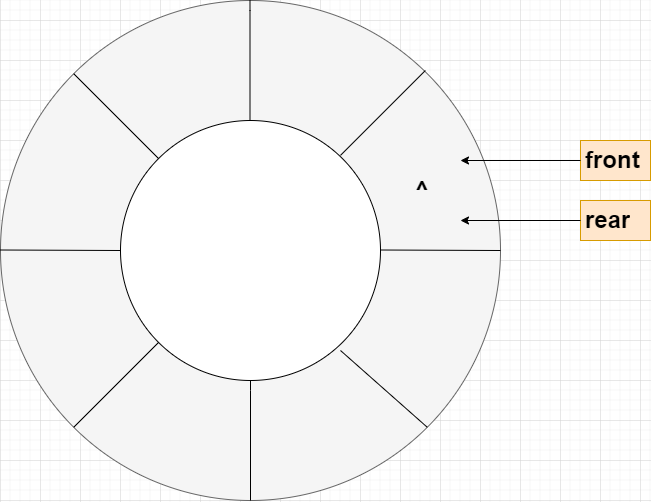``````//初始化
cir_queue *init(){
cir_queue *q = (cir_queue*)malloc(sizeof(cir_queue));
if(q==NULL){
exit(0);   //申请内存失败，退出程序
}
q->front=0;
q->rear=0;
return q;
}
``````

## 入队操作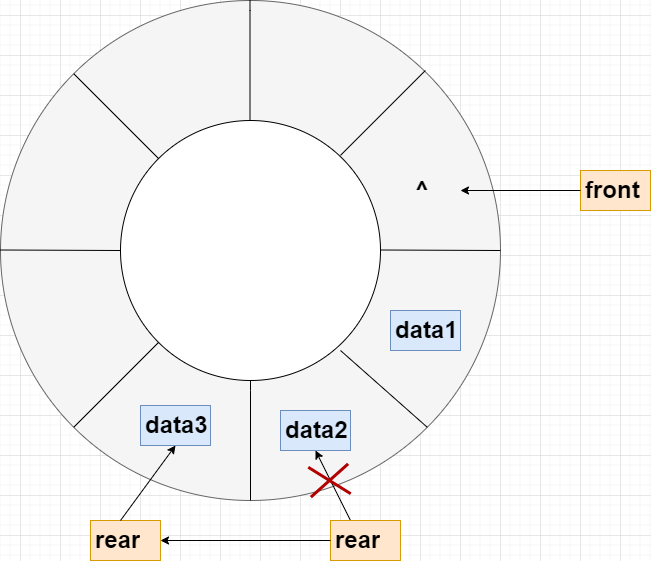入队操作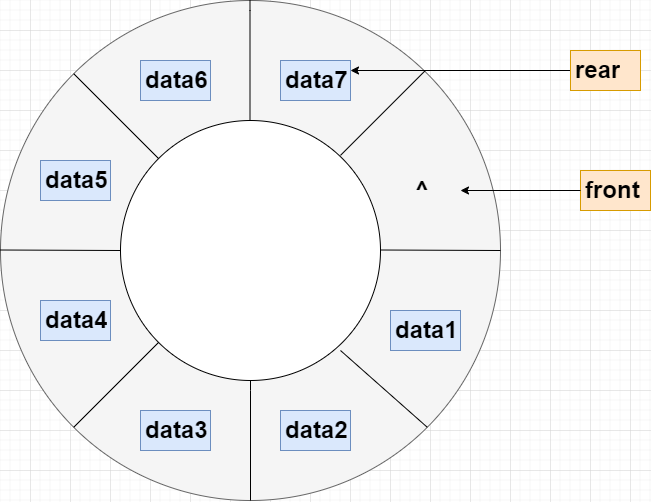队列已满

``````//入队操作push
void push(cir_queue *q,int data){
if((q->rear+1)%maxsize==q->front){
printf("溢出，无法入队\n");
return;
}else{
q->rear=(q->rear+1)%maxsize;
q->data[q->rear]=data;
}
}
``````

## 出队操作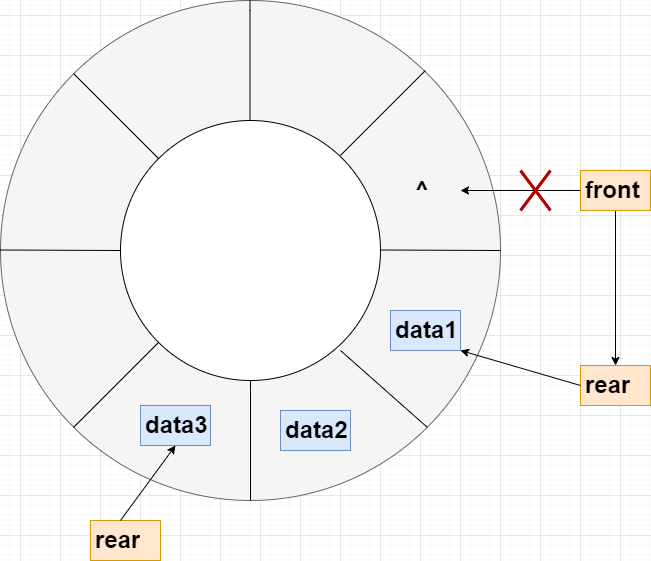出队操作

``````//出队操作pop
void pop(cir_queue *q){
if(q->rear==q->front){
printf("队列为空，无法出队\n");
return;
}else{
q->data[q->front]=0;
q->front=(q->front+1)%maxsize;
}
}
``````

## 遍历操作

``````//遍历队列
void print(cir_queue *q){
int i=q->front;
while(i!=q->rear){
i=(i+1)%maxsize;
printf("%d\t",q->data[i]);
}
printf("\n");       //记得换行
}
``````

## 关于队列的总结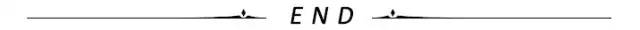blmius
1年前
MySQL:[Err] 1292 - Incorrect datetime value: ‘0000-00-00 00:00:00‘ for column ‘CREATE_TIME‘ at row 1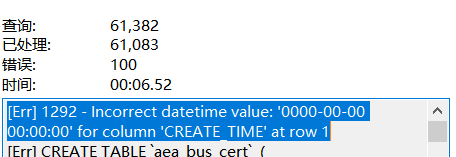Jacquelyn38
2年前
2020年前端实用代码段，为你的工作保驾护航Easter79
1年前
swap空间的增减方法
（1）增大swap空间去激活swap交换区：swapoff v /dev/vg00/lvswap扩展交换lv：lvextend L 10G /dev/vg00/lvswap重新生成swap交换区：mkswap /dev/vg00/lvswap激活新生成的交换区：swapon v /dev/vg00/lvswaphelloworld_34035044
11个月前

​在我们用皕杰报表工具设计填报报表时，如何在新增行里自动增加id呢？能新增整数排序id吗？目前可以在新增行里自动增加id，但只能用uuid函数增加UUID编码，不能新增整数排序id。uuid函数说明：获取一个UUID，可以在填报表中用来创建数据ID语法：uuid()或uuid(sep)参数说明：sep布尔值，生成的uuid中是否包含分隔符''，缺省为Wesley13
1年前
Java获得今日零时零分零秒的时间（Date型）
publicDatezeroTime()throwsParseException{    DatetimenewDate();    SimpleDateFormatsimpnewSimpleDateFormat("yyyyMMdd00:00:00");    SimpleDateFormatsimp2newSStella981
1年前
KVM调整cpu和内存Wesley13
1年前
mysql设置时区
mysql设置时区mysql\_query("SETtime\_zone'8:00'")ordie('时区设置失败，请联系管理员！');中国在东8区所以加8方法二：selectcount(user\_id)asdevice,CONVERT\_TZ(FROM\_UNIXTIME(reg\_time),'08:00','0Wesley13
1年前
Java日期时间API系列36
十二时辰，古代劳动人民把一昼夜划分成十二个时段，每一个时段叫一个时辰。二十四小时和十二时辰对照表：时辰时间24时制子时深夜11：00凌晨01：0023：0001：00丑时上午01：00上午03：0001：0003：00寅时上午03：00上午0Wesley13
1年前
00：Java简单了解Stella981
1年前Wesley13
1年前
MySQL部分从库上面因为大量的临时表tmp_table造成慢查询Wesley13
Lv1

1.2w

17

1.7k